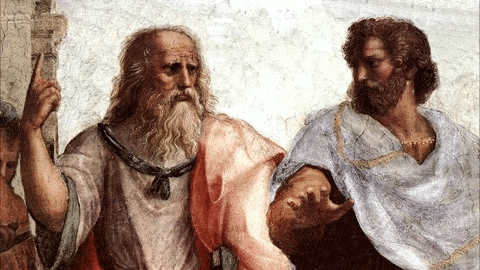# Adopt the Golden Rule Not Golden Ratio

Looking for perfect proportion or magic golden ratio to measure beauty? Well, remember the Golden Rule that beauty is in the eye of the beholder

Is everything chaos and chance?  The Greeks deeply influenced Western philosophy by elaborating a consistent system of numbers and proportions. Their aim was to measure order and harmony in human life, nature and the finest art.

## The meaning of numbers

Pythagoras first defined musical harmony, the intervals between notes, by the lengths of strings of the Lyre. While both are actually inversely proportional, he opted not to use the tone frequencies.  Interestingly, rhythm (Rhythmos) and number (Arithmos) share the same root: rheîn = to flow.

Plato then generalized the geometric proportion to all forms of aesthetic under a broader Metaphysic. For him, “Numbers are the highest degree of Knowledge.” Furthermore, “All these kinds of things received their shapes from the Ordering One, through the action of Ideas and Numbers.”

For the Pythagoreans, numbers have meaning. Five and the Ten are the most important ones.

• Five is the number of Love. You obtain five by uniting Two, the first even – female – number, and Three, the first odd greater than one – male – number.  Later, five became the symbol of humanity represented by the regular pentagon or pentagram.
• Ten, or the Decagon, symbolizes the universe. It was chosen as it is the sum of the first four numbers. Ten is equal to 1, the spiritual level, plus 2, the number for woman, plus 3, the number for man, plus 4, the four elements (Earth, Air, Water and Fire).Plato is dear to me, but dearer still is truth — Aristotle

## The math behind the Golden Ratio, or Divine Proportion

With the geometric representation comes the calculation of proportions. In turn, proportions allow for measurements and the idea, still very modern, that you can model the ultimate harmony of the world. Some intellectuals and mathematicians believe that a unique proportion exists in everything. This proportion is called the Golden Ratio, or sometime the Divine Proportion.

The Golden Ratio is calculated as follows:

• A geometrical proportion exists when “things” share similar relations, e.g. (a/b = c/d)
• Such proportion is called continuous when b = c. In this case, the proportion is reduced to three terms: (a/b = b/c).
• Assuming c = a+b provides a further simplification to 2 terms, i.e. (a/b = b/(a+b))
or (b/a)2 = (b/a)+1. (b/a) is the Golden Ratio and is usually represented by Φ.
• The solution to the [Φ2 – Φ – 1 = 0] quadratic equation is Φ = (1+√5)/2 = 1.618.A good decision is based on knowledge and not on numbers — Plato

## The Golden Rule is to treat others as one wants to be treated.

Approximation of this ratio has been discovered in some living forms. That is the case for the optimum angle between leaves or branches on a stem to obtain the maximum exposure to vertical light.

There are also claims that the human body integrates some Golden ratio proportions.  For instance, the bones of the fingers approximate a diminishing series of three terms (1, 1/Φ and 1/Φ2). As a result, the longest bone is approximately equaled to the sum of the two following ones.  However, there are no normalized way to make the measurement and, more often than not, the sizing is stretched to confirm the initial theory.

All this becomes very scary when the golden ratio is used to measure beauty, especially in human face.  Can a mathematical model really define beauty? Is a fixed proportion aesthetically pleasing?  Admittedly, we are again losing common sense and bow in front of this powerful cult that has survived millennia.

In reality, Beauty is in the eye of the beholder. You don’t want to judge others’ beauty in ways that you would not like your own to be judged!  Follow the golden rule, not the golden ratio.